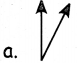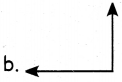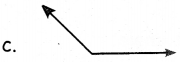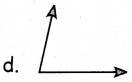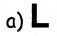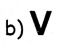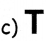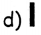Students can download 5th Maths Term 1 Chapter 1 Geometry Additional Questions and Answers, Notes, Samacheer Kalvi 5th Maths Guide Pdf helps you to revise the complete Tamilnadu State Board New Syllabus, helps students complete homework assignments and to score high marks in board exams.

## Tamilnadu Samacheer Kalvi 5th Maths Solutions Term 1 Chapter 1 Geometry Additional Questions

Write True or False.

Question 1.
Cuboid is covered by 6 rectangles _________
True

Question 2.
The height and slant height of the cone are unequal
True

Question 3.
The cube has 8 vertices
TrueQuestion 4.
Sphere has vertices and edges
False

Question 5.
Rectangle is a 2-D shape
True

Question 6.
The angle 90° is called as The right angle in AABC is
Right angle

Question 7.
In the following letters, which forms the acute angle?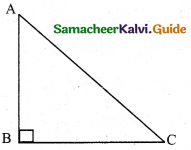(a) ∠A
(b) ∠B
(c) ∠C
(b) ∠BQuestion 8.
Hand of a clock at 3:00 shows ______ angle.
right

Question 9.
Circle the obtuse angle